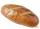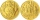# Negative in equation

2x + 3 + 7x = – 24, what is the value of x?

Result

x =  -3

#### Solution:

2x + 3 + 7x = -24

9x = -27

x = -3

Calculated by our simple equation calculator.

Leave us a comment of example and its solution (i.e. if it is still somewhat unclear...):

Showing 0 comments:Be the first to comment!#### To solve this example are needed these knowledge from mathematics:

Do you have a linear equation or system of equations and looking for its solution? Or do you have quadratic equation?

## Next similar examples:

1. Dropped sheetsThree consecutive sheets dropped from the book. The sum of the numbers on the pages of the dropped sheets is 273. What number has the last page of the dropped sheets?
2. Bed timeTiffany was 5 years old; her week night bedtime grew by ¼ hour each year. If, at age 18, her curfew time is 11pm, what was her bed time when she was 5 years old?
3. Bread5 of the same bread has the same weight as three bread and 4 kg of fruit. What weight has one bread?
4. Far countryIn a country far away, the value of 3 pesos is 12 centavos more than the value of 1 peso. How many centavos is 1 peso worth?
5. Find xSolve: if 2(x-1)=14, then x= (solve an equation with one unknown)
6. Equation 29Solve next equation: 2 ( 2x + 3 ) = 8 ( 1 - x) -5 ( x -2 )
7. Simple equationSolve for x: 3(x + 2) = x - 18
8. Unknown number 11That number increased by three equals three times itself?
9. Forest nurseryIn the forest nursery after winter, they found that 1/10 stems died out of them. For them, they land 193 new spruces. How many spruces are in the forest nursery?
10. Simple equationSolve the following simple equation: 2. (4x + 3) = 2-5. (1-x)
11. NormThree workers planted 3555 seedlings of tomatoes in one dey. First worked at the standard norm, the second planted 120 seedlings more and the third 135 seedlings more than the first worker. How many seedlings were standard norm?
12. TimeageSeven times of my age is 8 less than the largest two-digit number. How old I am?
13. Find unknownFind unknown numerator: 4/8 + _/8 = 1
14. Trees3/5 trees are apples, cherries are 1/3. 5 trees are pear. How many is the total number of trees?
15. TreesFrom the total number of trees in the orchard, there are two-fifths pearls and apples are three eighty. The rest of the trees are 9 ceremonial. How many trees are in the set?
16. CakesGrandmother baked cakes. Half of its was poppy, quarter with plum jam and 16 cheesecakes. How many cakes she baked in total?
17. FruitsAmy bought a basket of fruits 1/5 of them were apples,1/4 were oranges, and the rest were 33 bananas. How many fruits did she buy in all?